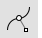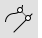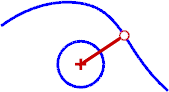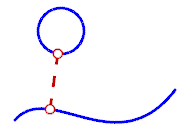ClosestPtCurve

Point Object >

Closest Point

The ClosestPt command creates a point object at the closest point from a specified location to a selected object or on two objects where they are closest to each other.Steps

1. Select the target object or objects.
2. Pick the base point for the closest point calculation.

Note

• The closest point on all of the objects is calculated.
• If more than one object is selected, the closest point will appear on only one object. To find the closest point on each object, use the ClosestPt command on each object individually.
• The distance is printed on the command line.
Command-line options

Object

Calculates the closest point between two objects.

To calculate the closest point to an object

• Select a curve or point object.
To select parts of objects, use sub-object selection.
The closest point from that object to the originally selected object is calculated.
Point objects are created at the closest points.
This is especially useful for testing two curve objects.CreateLine

Draws a line between the base point and the closest point on the object.

Type subcrv to select part of a curve as input.